Previous: 2.1 The Boltzmann Poisson Up: 2.1 The Boltzmann Poisson Next: 2.1.2 Poisson Equation

## 2.1.1 The Boltzmann Equation for Parabolic Energy Bands

For a distribution function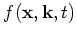where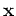is the position,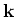is the wave vector and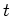is the time the Boltzmann equation for parabolic energy bands reads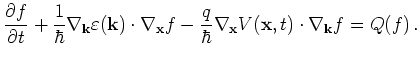(2.1)

Here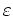is the given energy band diagram,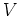is the electrostatic potential and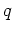is the carrier charge (negative for electrons). The independent variables are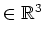,,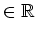.
We define the group velocity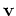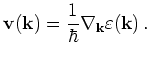(2.2)

For now we restrict ourselves to parabolic bands. Then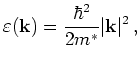(2.3)

with effective mass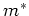and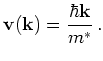(2.4)

The momentum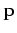is defined as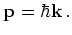(2.5)

Using the group velocitythe Boltzmann equation becomes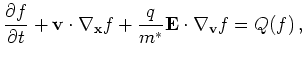(2.6)

where we introduced the electrical field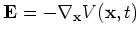.